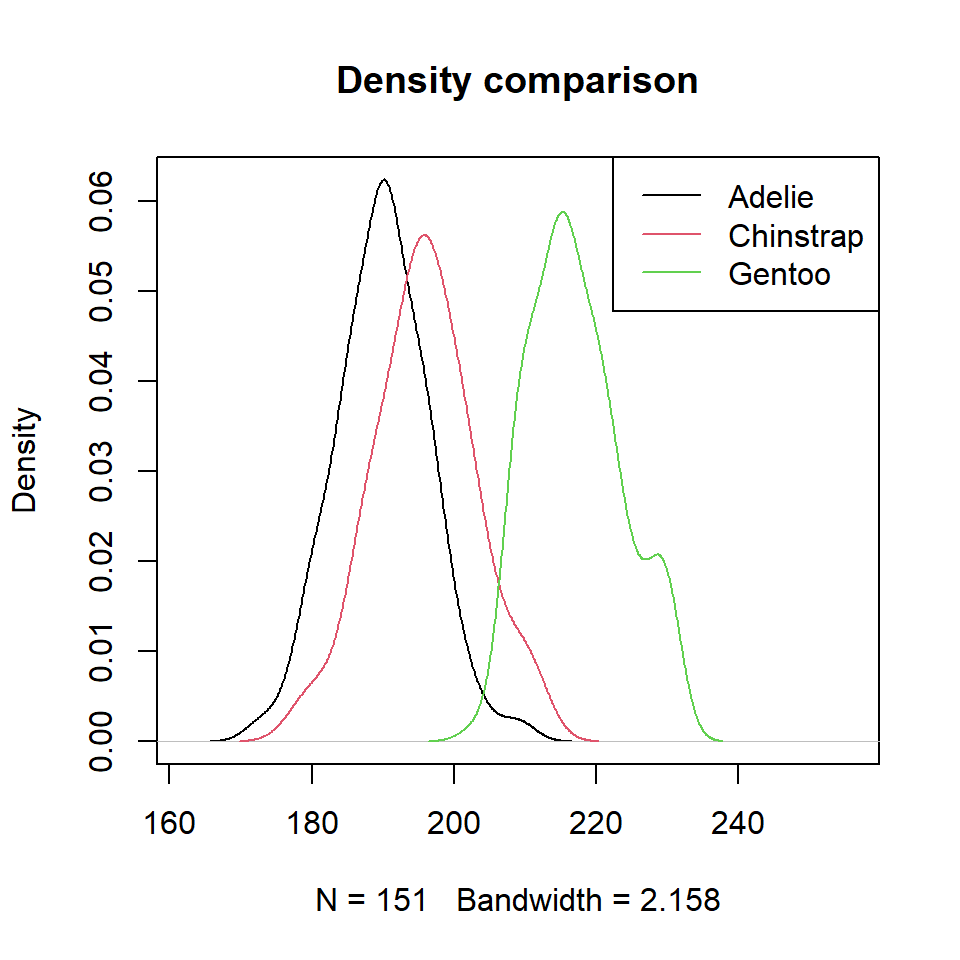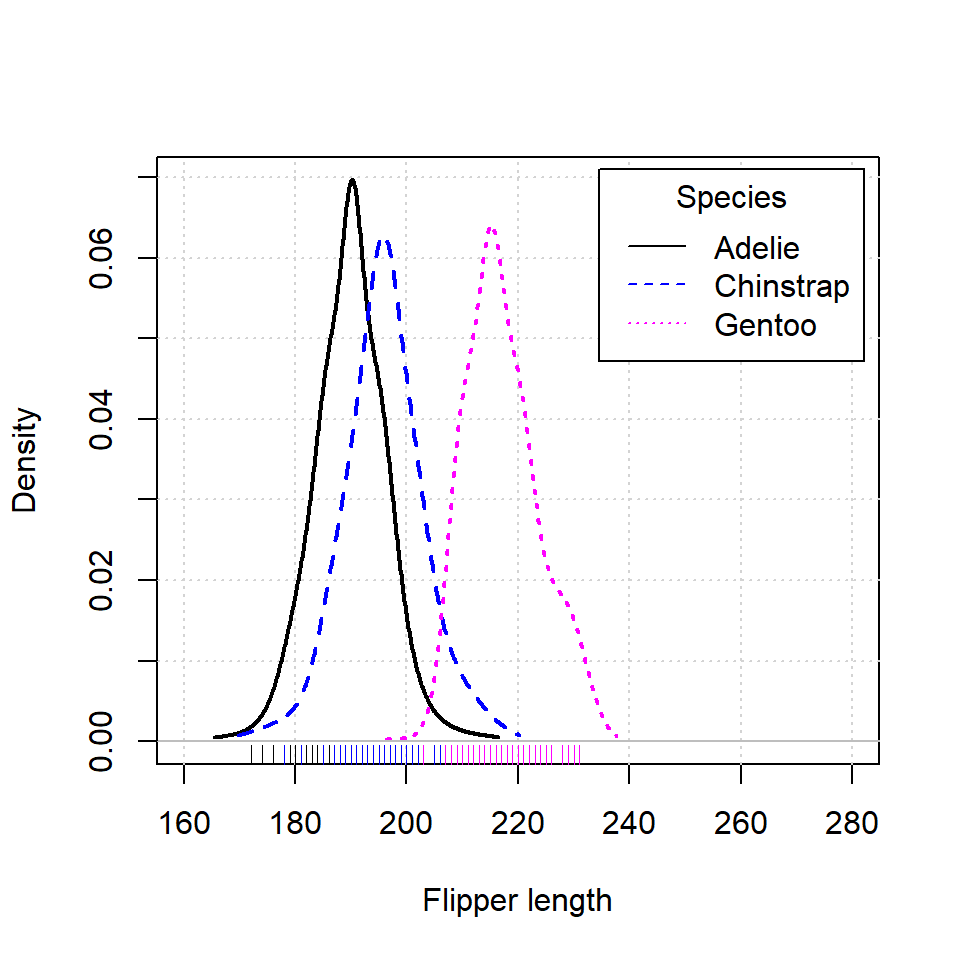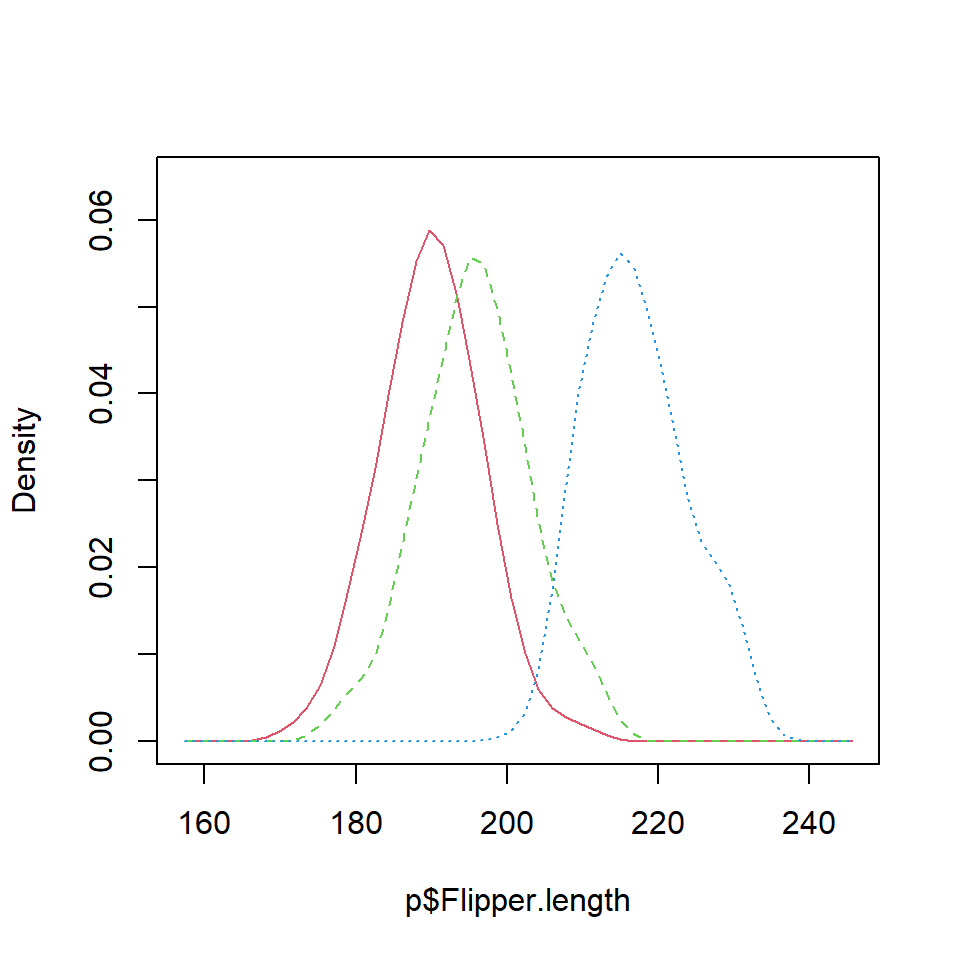# Density comparison chart in R

## Sample data

Consider the following data from `palmerpenguins` package, where the continuous variable is the flipper length of the pinguins and the categorial the penguin species.

``````# install.packages("palmerpenguins")
library(palmerpenguins)

x <- penguins\$flipper_length_mm
g <- as.factor(penguins\$species)

p <- data.frame(Flipper.length = x, species = g)
p <- na.omit(p)``````

## Density comparison with `lines`

It is possible to estimate the kernel density for each penguin species with the `tapply` function. Then, you can add the three densities to the same plot. Note that you can select a different kernel and a smoothing parameter than de defaults.

``````# install.packages("car")
library(car)

# Densities for each group
dens <- tapply(p\$Flipper.length, p\$species, density)

# Plot
plot(dens\$Adelie, xlim = c(min(p\$Flipper.length) - 10,
max(p\$Flipper.length) + 25),
main = "Density comparison")
lines(dens\$Chinstrap, col = 2)
lines(dens\$Gentoo, col = 3)

# Legend
legend("topright", legend = levels(p\$species),
lty = 1, col = 1:3)``````## The `densityPlot` functionAn alternative is using `densityPlot` from `car` package. Note that this function uses an adaptive-kernel estimate by default instead of a fixed-bandwidth kernel estimate. If you want the same estimation of the previous section set `method = "kernel"` as argument.

``````# install.packages("car")
library(car)

densityPlot(p\$Flipper.length ~ p\$species,
legend = list(title = "Species"),
xlab = "Flipper length",
xlim = c(160, 280))``````

## The `sm.density.compare` function

Other option is using the `sm.density.compare` function from `sm` library. If you set `model = "equal"` a permutation test of equality will be computed and boostrap bands will be displayed if the number of groups is two.

``````# install.packages("sm")
library(sm)

sm.density.compare(x = p\$Flipper.length,
group = p\$species,
model = "equal")``````A journey of imagination, exploration, and beautiful data visualizations